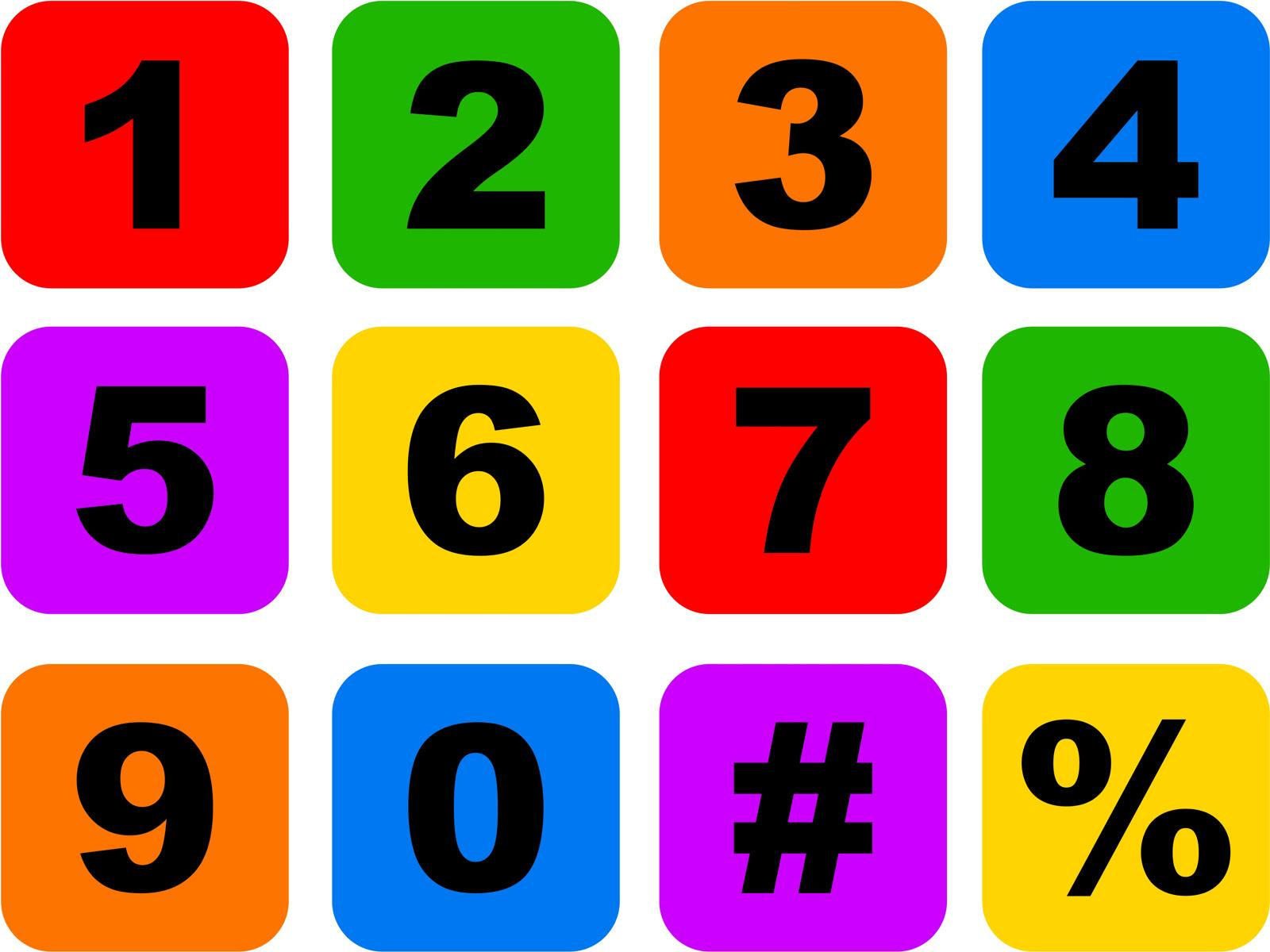# 2nd smallest number puzzle

963.2K Views

There is 38 numbers. What is the least number of comparison needed to find the 2nd smallest out of them?Answer: 52 comparisons which is also the worst case

Here’s how the algorithm works:

1. Divide the 38 numbers into pairs, and find the smallest number in each pair. This takes 19 comparisons.
2. Take the 19 smallest numbers and pair them up again, finding the smallest number in each pair. This takes 18 comparisons.
3. Take the 9 smallest numbers and pair them up again, finding the smallest number in each pair. This takes 8 comparisons.
4. Take the 5 smallest numbers and pair them up again, finding the smallest number in each pair. This takes 4 comparisons.
5. Take the 3 smallest numbers and pair them up again, finding the smallest number in each pair. This takes 2 comparisons.
6. Take the 2 remaining numbers and find the smallest one. This takes 1 comparison.

Adding up the comparisons from each step gives us a total of 19+18+8+4+2+1=52 comparisons. However, in the worst case scenario, the two smallest numbers are always paired up in the last step.